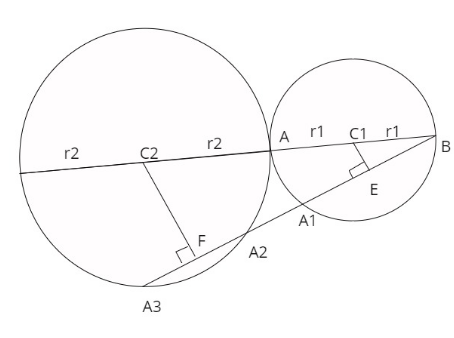QUESTION

# Let ${C_{1,}}{C_2}$ be two circles touching each other externally at the point A and let AB be the diameter of the circle ${C_1}$. Draw a secant $B{A_3}$ to circle ${C_2}$, intersecting to circle ${C_1}$ at a point ${A_1}$($\ne A)$ , and circle ${C_2}$ at points ${A_2}$ and ${A_3}$ .If $B{A_1}$=2, $B{A_2} = 3$ and $B{A_3} = 4$ ,then the radii of the circles ${C_1}$ and ${C_2}$ are respectively.

Hint: On drawing the figure with the data given, we get two triangles, let us prove that those triangles are similar and solve the problem further.On drawing the figure with respect to the given data, we get two triangles here,
That is $\vartriangle B{C_1}E$ and $\vartriangle B{C_2}F$
Now ,let us compare these two triangles
So, in $\vartriangle B{C_1}E$ and $\vartriangle B{C_2}F$,we can compare and write
$\angle {C_1}EB = \angle {C_2}FB = {90^ \circ } \\ \angle {C_1}BE = \angle {C_2}BF = {\text{common angle}} \\ \angle B{C_1}E = \angle B{C_2}F = {\text{remaining angle}} \\ \\$
So, therefore we can write $\vartriangle B{C_1}E$ and $\vartriangle B{C_2}F$ are similar to each other(from AA postulate)

So , from this we can write
$\dfrac{{B{C_1}}}{{B{C_2}}} = \dfrac{{BE}}{{BF}}$ (Corresponding sides of two similar triangles are proportional)
On comparing with the figure, we can substitute the value of $B{C_1}$ ,$B{C_2}$ , BE and BF as follows
$\dfrac{{{r_1}}}{{{r_1} + {r_1} + {r_2}}} = \dfrac{1}{{3 + \dfrac{1}{2}}}$ (Since, centre ${C_1}$ and ${C_2}$ bisects $B{A_{1,}}{A_2},{A_3}{\text{ with }} \bot {\text{ at E,F}}$ )
On simplifying this further we get $3{r_1} = 2{r_2}$
$\Rightarrow {r_2} = \dfrac{3}{2}{r_1}$
Also, if we consider P as an external point from which a secant is cutting a circle at point A,B then we can write $PA \times PB$ =constant
So, we can write $B{C_1} \times B{C_2} = BE \times BF$
Let us substitute the values of $B{C_1},B{C_2}$ ,BE ,BF in the equation, so we get
$\Rightarrow 4{r_1}({r_1} + {r_2}) = 4 \times 3 \\ \Rightarrow {r_1}^2 + \dfrac{3}{2}{r_1}^2 = 3 \\ \Rightarrow {r_1}^2 = \dfrac{6}{5} \\ \Rightarrow {r_1} = \dfrac{{\sqrt {30} }}{5} \\ \Rightarrow {r_{2 = }}\dfrac{3}{2}\dfrac{{\sqrt {30} }}{5} = \dfrac{{3\sqrt {30} }}{{10}} \\$
So , we get the radius of circle ${C_1}$ =$\dfrac{{\sqrt {30} }}{5}$ and radius of circle ${C_2} = \dfrac{{3\sqrt {30} }}{{10}}$

Note: The secant of a circle is the line that intersects the circle at minimum two points, also if a perpendicular is drawn from the centre of the circle to the secant then it bisects it. Take the data properly from the diagram.